# 410. [V] The transformation T.1.1: R3 R3, Tk,1,1 (u, v, w) = (x, y, z) of...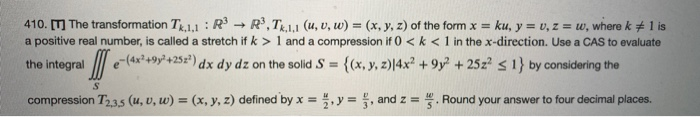410. [V] The transformation T.1.1: R3 R3, Tk,1,1 (u, v, w) = (x, y, z) of the form x = ku, y = 0, z = w, wherek #1 is a positive real number, is called a stretch if k > 1 and a compression if 0 <k < 1 in the x-direction. Use a CAS to evaluate the integral e-(4x2+9y?+252) dx dy dz on the solid S = {(x, y, z)|4x² +9y2 + 25z< 1} by considering the compression T2,3,5 (u, v, w) = (x, y, z) defined by x = , y = , and z = . Round your answer to four decimal places.

.

please thumbs up for this solution..thanks..

.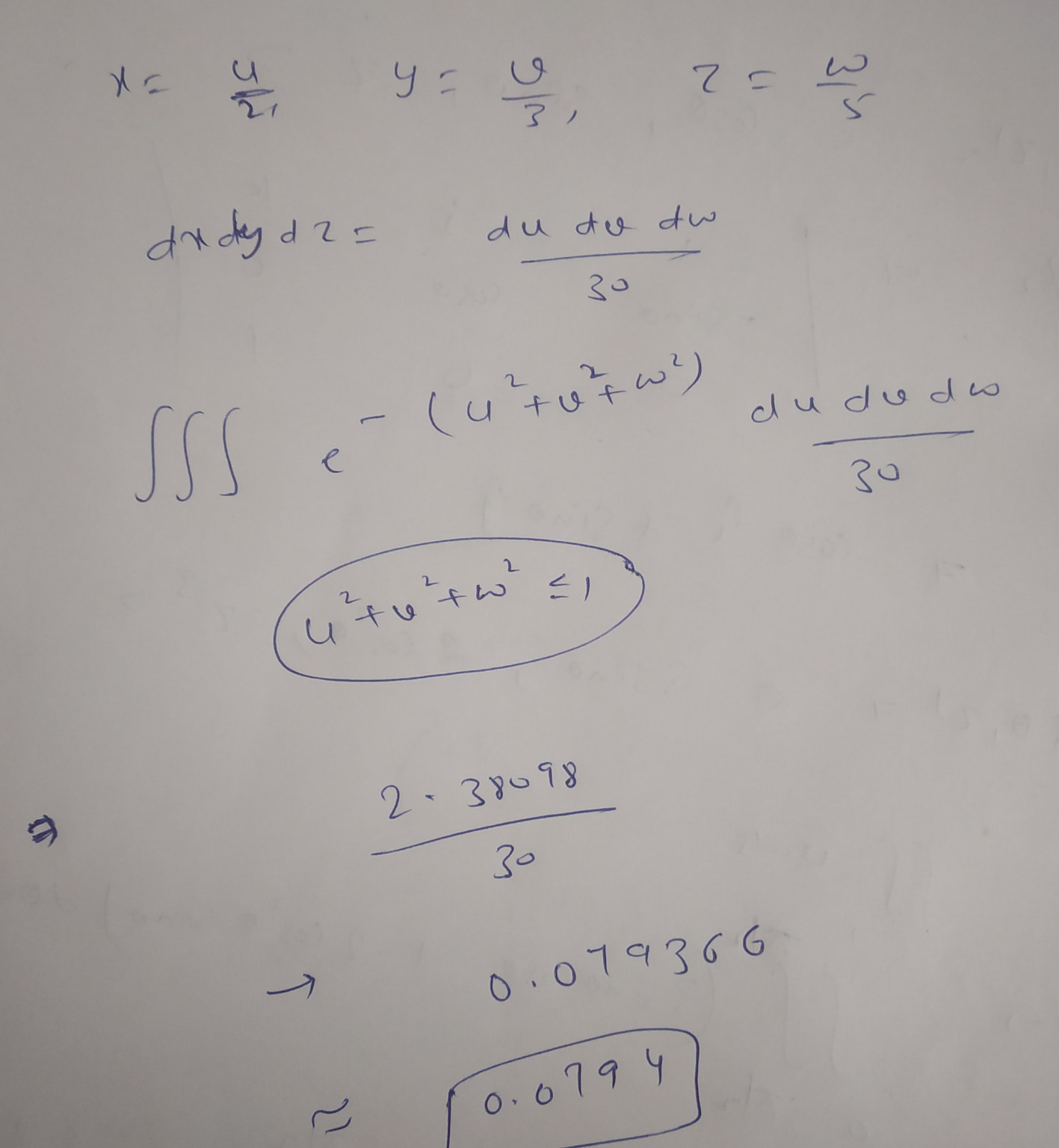##### Add Answer of: 410. [V] The transformation T.1.1: R3 R3, Tk,1,1 (u, v, w) = (x, y, z) of...
Similar Homework Help Questions
• ### For U = {q, r, s, t, u, v, w, x, y, z} A = {q, s, u, w, y} B = {q, s, y, z} C = {v, w, x, y, z} identify the following

For U = {q, r, s, t, u, v, w, x, y, z}A = {q, s, u, w, y}B = {q, s, y, z}C = {v, w, x, y, z}identify the following.5) CA) {v, w, x, y, z}B) {q, r, s, t, u}C) { }D) {v, z}

• ### transformation. Perform the mappings of lines x- 2 and y 3 under the transformation w = z2 where z = x + iy. Compute the angles between the curves in the u-v plane at the points of intersection. Henc...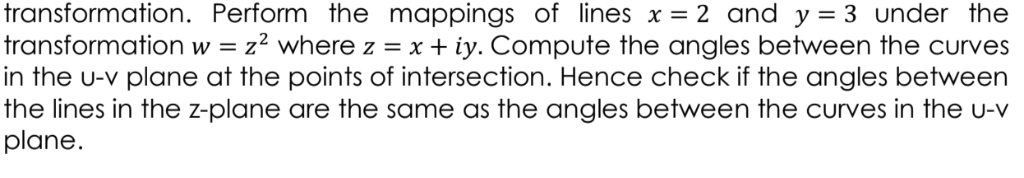transformation. Perform the mappings of lines x- 2 and y 3 under the transformation w = z2 where z = x + iy. Compute the angles between the curves in the u-v plane at the points of intersection. Hence check if the angles between the lines in the z-plane are the same as the angles between the curves in the u-v plane transformation. Perform the mappings of lines x- 2 and y 3 under the transformation w = z2 where...

• ### let U= {q,r,s,t,u,v,w,x,y,z}; A= {q,s,u,w,y};and C={v,w,x,y,z,}; list the members of the indicated set , using set braces A'u B A

4.let U= {q,r,s,t,u,v,w,x,y,z}; A= {q,s,u,w,y};and C={v,w,x,y,z,}; list the members of the indicated set , using set braces A'u B A.{Q,R,S,T,V,X,Y,Z} B.{S,U,W} C.{R,S,T,U,V,W,X,Z} D.{Q,S,T,U,V,W,X,Y}

• ### Define an equivalence relation on R by (x,y,z) ∼ (u,v,w) whenever x +y +z = u...

Define an equivalence relation on R by (x,y,z) ∼ (u,v,w) whenever x +y +z = u +v +w . Describe the equivalence classes.

• ### z+1 The linear fractional transformation shown below is related to w = u + jv and...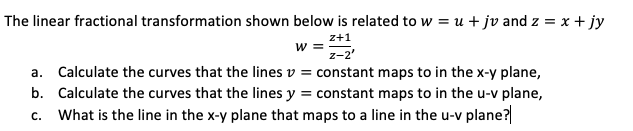z+1 The linear fractional transformation shown below is related to w = u + jv and z = x + jy w= z-2' a. Calculate the curves that the lines v = constant maps to in the x-y plane, b. Calculate the curves that the lines y = constant maps to in the u-v plane, c. What is the line in the x-y plane that maps to a line in the u-v plane?

• ### (1 point) The equations define u(x, y) and v(x, y) in terms of x and y near the point (x, y)-(1,1) and (u, v)-(1,1). Co...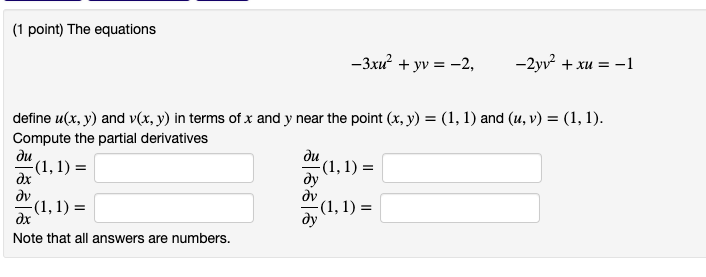(1 point) The equations define u(x, y) and v(x, y) in terms of x and y near the point (x, y)-(1,1) and (u, v)-(1,1). Compute the partial derivatives ди du dx 0v dy dv ду Note that all answers are numbers. (1 point) The equations define u(x, y) and v(x, y) in terms of x and y near the point (x, y)-(1,1) and (u, v)-(1,1). Compute the partial derivatives ди du dx 0v dy dv ду Note that all answers...

• ### Transformation T:R' -»R',T(x,y, z) = (x+y,x-z)nd v=< 1,-1,2 > 8. ar iven B <1,1,1>,< 1,0,1 >&...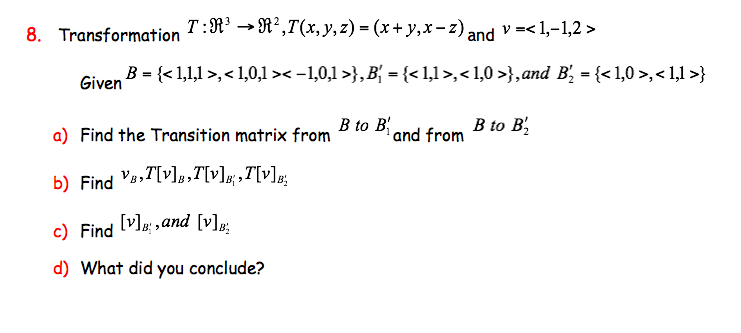Transformation T:R' -»R',T(x,y, z) = (x+y,x-z)nd v=< 1,-1,2 > 8. ar iven B <1,1,1>,< 1,0,1 ><-1,0,1>},B^ = {<1,1>,<1,0 >},and B, = {<1,0>,< 1,1>} B to Biand from B to B2 a) Find the Transition matrix from b) Find v],T[v];,7[v] c) Find v,and [v]p d) What did you conclude? Transformation T:R' -»R',T(x,y, z) = (x+y,x-z)nd v= 8. ar iven B ,},B^ = {,},and B, = {,} B to Biand from B to B2 a) Find the Transition matrix from b) Find...

• ### Question 2 Let U = {q, r, s, t, u, v, w, x, y, z} C...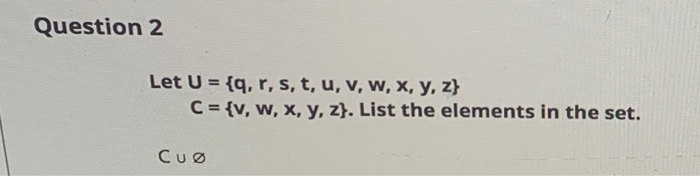Question 2 Let U = {q, r, s, t, u, v, w, x, y, z} C = {v, w, x, y, z}. List the elements in the set. CU

• ### A {r,u,v,y} B {r,v,w,x} C {r,u,w,z} t and s are on the outsides

A {r,u,v,y}B {r,v,w,x}C {r,u,w,z} t and s are on the outsides.(a) (A B) Cc(b) (A B C)c

• ### Lets U = {q,r,s,t,u,w,x,y,z}; A={q,s,u,w,y}; B={q,s,y,z} and C ={v,w,x,y,z} List the members of the indicated set, using set braces

Lets U = {q,r,s,t,u,w,x,y,z}; A={q,s,u,w,y}; B={q,s,y,z} and C ={v,w,x,y,z} List the members of the indicated set, using set braces.

Free Homework App